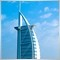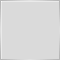# iCustom() - How to use it?1644

So I am wanting to incorporate ADR into my algorithm and have picked up a public indicator rather than spending time writing it myself. Does the trick for what I am after.

```//+------------------------------------------------------------------+
//|                                           Daily Range PeterE.mq4 | //< Credit to PeterE
//+------------------------------------------------------------------+

#property indicator_chart_window

input int      NumOfDays             = 10;
extern string   FontName              = "Courier New";
extern int      FontSize              = 10;
extern color    FontColor             = White;
extern int      Window                = 0;
extern int      Corner                = 0;
extern int      HorizPos              = 5;
extern int      VertPos               = 20;

double pnt;
int    dig;
string objname = "*DRPE";

//+------------------------------------------------------------------+
int init()  {
//+------------------------------------------------------------------+
pnt = MarketInfo(Symbol(),MODE_POINT);
dig = MarketInfo(Symbol(),MODE_DIGITS);
if (dig == 3 || dig == 5) {
pnt *= 10;
}
ObjectCreate(objname,OBJ_LABEL,Window,0,0);
return(0);
}

//+------------------------------------------------------------------+
int deinit()  {
//+------------------------------------------------------------------+
ObjectDelete(objname);
return(0);
}

//+------------------------------------------------------------------+
int start()  {
//+------------------------------------------------------------------+
int c=0;
double sum=0;
for (int i=1; i<Bars-1; i++)  {
double hi = iHigh(NULL,PERIOD_D1,i);
double lo = iLow(NULL,PERIOD_D1,i);
datetime dt = iTime(NULL,PERIOD_D1,i);
if (TimeDayOfWeek(dt) > 0 && TimeDayOfWeek(dt) < 6)  {
sum += hi - lo;
c++;
if (c>=NumOfDays) break;
} }
hi = iHigh(NULL,PERIOD_D1,0);
lo = iLow(NULL,PERIOD_D1,0);
if (i>0 && pnt>0)  {
string objtext = "ADR = " + DoubleToStr(sum/c/pnt,1) + "  (" + c + " days)     Today = " + DoubleToStr((hi-lo)/pnt,1);
ObjectSet(objname,OBJPROP_CORNER,Corner);
ObjectSet(objname,OBJPROP_XDISTANCE,HorizPos);
ObjectSet(objname,OBJPROP_YDISTANCE,VertPos);
ObjectSetText(objname,objtext,FontSize,FontName,FontColor);
}
return(0);
}
```
`double ADR = iCustom(NULL,low,"Average Daily Range", 0, 0);`

With regards to iCustom, I am wanting to know when price exceeds the days ADR. I'm trying to understand what value is being returns because at the moment I do not understand where it is derived from if I print double "ADR".24689

 DomGilberto:  With regards to iCustom, I am wanting to know when price exceeds the days ADR. I'm trying to understand what value is being returns because at the moment I do not understand where it is derived from if I print double "ADR".`double ADR = iCustom(NULL,low,"Average Daily Range", 0, 0);` ICustom read the specified buffer at the specified shift. Nothing more. Detailed explanation of iCustom - MQL4 forum Your indicator doesn't have any buffers, you can't use iCustom.1644

Ok thanks for your reply (quite amazing how you pull up old threads so quickly like that!) :)

Anyway, I've had a read and I think am getting confused with the array part...

This is what I have so far:

```// ---- indicator ----

input int      NumOfDays             = 10;
extern string   FontName              = "Courier New";
extern int      FontSize              = 10;
extern color    FontColor             = White;
extern int      Window                = 0;
extern int      Corner                = 0;
extern int      HorizPos              = 5;
extern int      VertPos               = 20;

double pnt;
int    dig;
string objname = "*DRPE";

double   ADR[];

//+------------------------------------------------------------------+
int init()  {
//+------------------------------------------------------------------+
SetIndexBuffer(0,ADR);

...

...

//+------------------------------------------------------------------+
int start()  {
//+------------------------------------------------------------------+
int c=0;
double sum=0;
for (int i=1; i<Bars-1; i++)  {
double hi = iHigh(NULL,PERIOD_D1,i);
double lo = iLow(NULL,PERIOD_D1,i);
datetime dt = iTime(NULL,PERIOD_D1,i);
if (TimeDayOfWeek(dt) > 0 && TimeDayOfWeek(dt) < 6)  {
sum += hi - lo;
c++;
if (c>=NumOfDays) break;
} }
hi = iHigh(NULL,PERIOD_D1,0);
lo = iLow(NULL,PERIOD_D1,0);
if (i>0 && pnt>0)  {
string objtext = "ADR = " + DoubleToStr(sum/c/pnt,1) + "  (" + c + " days)     Today = " + DoubleToStr((hi-lo)/pnt,1); // average on the left equation. Todays range on the right
ObjectSet(objname,OBJPROP_CORNER,Corner);
ObjectSet(objname,OBJPROP_XDISTANCE,HorizPos);
ObjectSet(objname,OBJPROP_YDISTANCE,VertPos);
ObjectSetText(objname,objtext,FontSize,FontName,FontColor);

ADR = (hi-lo)/pnt; //<< Obviously not correct...

}
return(0);
}

```
```// ------ EA -------------//

// Global

//+--------------------------
input int NumOfDays            = 10;
string   FontName              = "Courier New";
int      FontSize              = 10;
color    FontColor             = White;
int      Window                = 0;
int      Corner                = 0;
int      HorizPos              = 5;
int      VertPos               = 20;
#define  TodaysRange 0
//+-------------------------

...

...

double ADR = iCustom(NULL,low,"Average Daily Range",NumOfDays,FontName,FontSize,FontColor,Window,Corner,HorizPos,VertPos,TodaysRange,0);

...

```

Now obviously when trying to compile the indicator, I am getting "Invalid array access".

I don't think I am understanding the array part...?1644

Also, I could just technically build a function within my EA instead of using iCustom()... might be easier and more efficient... or not?17374

DomGilberto:

Ok thanks for your reply (quite amazing how you pull up old threads so quickly like that!) :)

Anyway, I've had a read and I think am getting confused with the array part...

This is what I have so far:

Now obviously when trying to compile the indicator, I am getting "Invalid array access".

I don't think I am understanding the array part...?

ADR is an array so when you give it a value . . . = (hi-lo)/pnt;  you need to specify which cell in the array the value is for . . .  e.g.

`ADR[i] = (hi-lo)/pnt;`1644

Ok thanks. Am I doing the iCustom part right on the actual EA? I assume that as long as I have this indicator compiled on the same platform iCustom can be called and used during ST?

At the moment the value being returned in the print looks like this:

```2014.10.09 09:12:31.359 2004.01.02 03:00  TF - v2.7 - Sandbox - MO EURUSD,H1: ADR is == 2147483647.0
```
I am trying to return the ADR value for the given day on EURUSD...17374

DomGilberto:
Ok thanks. Am I doing the iCustom part right on the actual EA?

Possibly not,  what is  low  ?  is it an int that represents the timeframe ?

`double ADR = iCustom(NULL,   low,   "Aver`1644

My mistake, it's all working now. Thanks for your help as usual (and of course WHRoeder for pointing out that valuable thread!)

(low = lowest timeframe. So yeah, it's int 60)433

What was the solution? I have the same problem:

`iCustom(instrument, period, "ADR", 7, 0, 1);`

returns 2147483647.0Moderator
2315

ShariffS:

What was the solution? I have the same problem:

returns 2147483647.0

EMPTY_VALUE24689

 ShariffS: What was the solution? I have the same problem: returns 2147483647.0`iCustom(instrument, period, "ADR", 7, 0, 1);` Do you have an indicator adr.ex4? Dom's was named "Average Daily Range.ex4"Dom was trying to add a buffer, but never added the #indicator_buffers and #indicator_separate_window